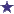# Ratio/Concentration: 983mg in 250ml bag - 783mg is needed, how much volume do I remove?

by Veronica
(Miami Beach, FL, USA)Ratio Volume / Concentration Question -- How much volume needs to be removed?

I have 983mg in a 250 ml bag I need 783mg how much volume do I remove?

### Comments for Ratio/Concentration: 983mg in 250ml bag - 783mg is needed, how much volume do I remove?

Average RatingRatingWithdraw 51 ml from the bag... by: Anonymous The previous answer is only partially right. You just didn't go far enough. 199 ml is what you should be left with in the bag (to get the correct strength), not withdraw from the bag. See for yourself and follow the following process: Set up the ratio equation: (what we have) 983(mg strength):250(ml volume) = (what we need) 783(mg strength): X (ml volume) Now, divide 783 by 983 = 0.796 Then multiply 0.796 by 250 mL and the answer is: X=199mL. Finally, we subtract 199 from 250… 250-199= 51 Withdraw 51 ml from the bag.

 RatingRemove 199mL from the Bag for 783mg by: David 983:250 = 783: X divide 783 by 983 = 0.796 then multiply 0.796 by 250mL and the answer is: X=199mL